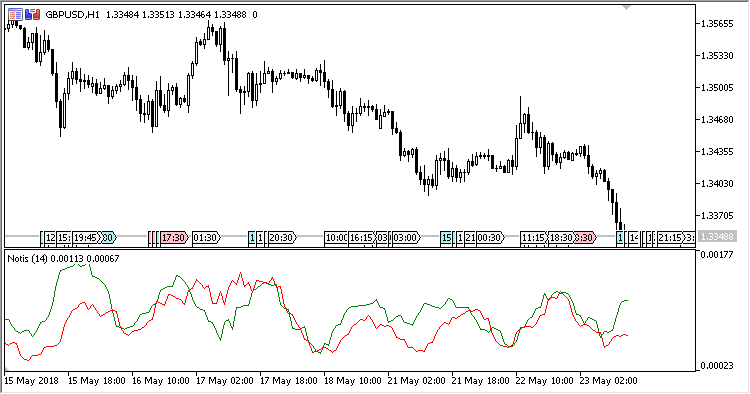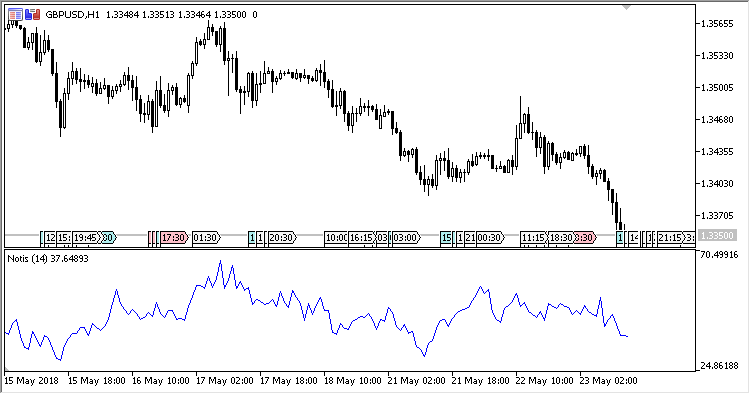# Notis – indicator for MetaTrader 5

Oscillator Notis% V measures the market volatility, based on the difference between the intraday High and Low.

Volatility is high, when the difference between lines Plus and Minus is large; volatility is low, when the difference is small.

The indicator can work in two modes of calculating and data providing:

1. Cumulative mode. In this mode, the indicator behaves as a normal oscillator having one line. In this mode, the indicator chart can also be inverted.
2. Non-cumulative mode (by default). In this mode, the indicator shows two line: Plus and Minus. The difference between the lines points to the volatility level of the market. Crossing – direction.

The indicator has four input parameters:

• Period – calculation period;
• Method – averaging method;
• Cumulative mode – cumulative mode (switch Yes/No);
• Inverse in cumulative mode – inverting the indicator chart in cumulative mode (switch Yes/No).

Calculations:

`NOTIS[i] = 100*Plus[i] / (Plus[i] + Minus[i])`

where:

```Plus = Moving Average(P, Period, Method)
Minus = Moving average(M, Period, Method)
P[i] = High[i] - Close[i]
M[i] = Close[i] - Low[i]
```Related Posts

Fig.1. mode by default

Fig.2. Cumulative mode without invertingFig.3. Cumulative mode with inverting